Home
Hostname: page-component-66d7dfc8f5-vmgfl Total loading time: 3.849 Render date: 2023-02-08T16:10:36.573Z Has data issue: true Feature Flags: { "useRatesEcommerce": false } hasContentIssue trueCompositio Mathematica

# On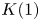$K(1)$-local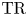$\mathrm {TR}$

Published online by Cambridge University Press:  04 May 2021

## Abstract

We discuss some general properties of$\mathrm {TR}$ and its$K(1)$-localization. We prove that after$K(1)$-localization,$\mathrm {TR}$ of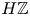$H\mathbb {Z}$-algebras is a truncating invariant in the Land–Tamme sense, and deduce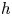$h$-descent results. We show that for regular rings in mixed characteristic,$\mathrm {TR}$ is asymptotically$K(1)$-local, extending results of Hesselholt and Madsen. As an application of these methods and recent advances in the theory of cyclotomic spectra, we construct an analog of Thomason's spectral sequence relating$K(1)$-local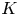$K$-theory and étale cohomology for$K(1)$-local$\mathrm {TR}$.

## MSC classification

Type
Research Article
Information
Compositio Mathematica , May 2021 , pp. 1079 - 1119

## Access options

Get access to the full version of this content by using one of the access options below. (Log in options will check for institutional or personal access. Content may require purchase if you do not have access.)

## Footnotes

This work was done while the author was a Clay Research Fellow.

## References

Angelini-Knoll, G. and Quigley, J. D., The Segal conjecture for topological Hochschild homology of Ravenel spectra, J. Homotopy Relat. Struct. 16 (2021), 4160.CrossRefGoogle Scholar
Antieau, B., Mathew, A., Morrow, M. and Nikolaus, T., On the Beilinson fiber square, Preprint (2020), arXiv:2003.12541.Google Scholar
Antieau, B. and Nikolaus, T., Cartier modules and cyclotomic spectra, J. Amer. Math. Soc. 34 (2021), 178.CrossRefGoogle Scholar
Aoki, K., Tensor triangular geometry of filtered objects and sheaves, Preprint (2020), arXiv:2001.00319.Google Scholar
Ausoni, C. and Rognes, J., Algebraic$K$-theory of topological$K$-theory, Acta Math. 188 (2002), 139.10.1007/BF02392794CrossRefGoogle Scholar
Ayala, D. and Francis, J., Factorization homology of topological manifolds, J. Topol. 8 (2015), 10451084.CrossRefGoogle Scholar
Ayala, D., Francis, J. and Tanaka, H. L., Factorization homology of stratified spaces, Selecta Math. (N.S.) 23 (2017), 293362.10.1007/s00029-016-0242-1CrossRefGoogle Scholar
Bhatt, B., Clausen, D. and Mathew, A., Remarks on$K (1)$-local$K$-theory, Selecta Math. (N.S.) 26 (2020), Paper No. 39.CrossRefGoogle Scholar
Bhatt, B. and Mathew, A., The arc-topology, Duke Math. J., to appear. Preprint (2018), arXiv:1807.04725.Google Scholar
Bhatt, B., Morrow, M. and Scholze, P., Integral$p$-adic Hodge theory, Publ. Math. Inst. Hautes Études Sci. 128 (2018), 219397.CrossRefGoogle Scholar
Bhatt, B., Morrow, M. and Scholze, P., Topological Hochschild homology and integral$p$-adic Hodge theory, Publ. Math. Inst. Hautes Études Sci. 129 (2019), 199310.CrossRefGoogle Scholar
Bhatt, B. and Scholze, P., The pro-étale topology for schemes, Astérisque 369 (2015), 99201.Google Scholar
Bhatt, B. and Scholze, P., Projectivity of the Witt vector affine Grassmannian, Invent. Math. 209 (2017), 329423.CrossRefGoogle Scholar
Bhatt, B. and Scholze, P., Prisms and prismatic cohomology, Preprint (2019), arXiv:1905.08229.Google Scholar
Blumberg, A. J., Gepner, D. and Tabuada, G., A universal characterization of higher algebraic$K$-theory, Geom. Topol. 17 (2013), 733838.CrossRefGoogle Scholar
Bökstedt, M., Bruner, R. R., Lunøe-Nielsen, S. and Rognes, J., On cyclic fixed points of spectra, Math. Z. 276 (2014), 8191.10.1007/s00209-013-1187-0CrossRefGoogle Scholar
Bökstedt, M., Hsiang, W. C. and Madsen, I., The cyclotomic trace and algebraic$K$-theory of spaces, Invent. Math. 111 (1993), 465539.CrossRefGoogle Scholar
Bökstedt, M. and Madsen, I., Topological cyclic homology of the integers,$K$-theory (Strasbourg, 1992), Astérisque 226 (1994), 7–8, 57–143.Google Scholar
Brun, M., Filtered topological cyclic homology and relative$K$-theory of nilpotent ideals, Algebr. Geom. Topol. 1 (2001), 201230.CrossRefGoogle Scholar
Česnavičius, K. and Scholze, P., Purity for flat cohomology, Preprint (2019), arXiv:1912.10932.Google Scholar
Clausen, D. and Mathew, A., Hyperdescent and étale K-theory, Invent. Math., to appear. Preprint (2019), arXiv:1905.06611.Google Scholar
Clausen, D., Mathew, A. and Morrow, M.,$K$-theory and topological cyclic homology of henselian pairs, J. Amer. Math. Soc. 34 (2021), 411473.Google Scholar
Clausen, D., Mathew, A., Naumann, N. and Noel, J., Descent in algebraic$K$-theory and a conjecture of Ausoni–Rognes, J. Eur. Math. Soc. (JEMS) 22 (2020), 11491200.CrossRefGoogle Scholar
Crabb, M. and James, I., Fibrewise homotopy theory, Springer Monographs in Mathematics (Springer, London, 1998).CrossRefGoogle Scholar
Dugger, D., Hollander, S. and Isaksen, D. C., Hypercovers and simplicial presheaves, Math. Proc. Cambridge Philos. Soc. 136 (2004), 951.CrossRefGoogle Scholar
Dundas, B. I., Goodwillie, T. G. and McCarthy, R., The local structure of algebraic K-theory, Algebra and Applications, vol. 18 (Springer, London, 2013).Google Scholar
Gabber, O.,$K$-theory of Henselian local rings and Henselian pairs, in Algebraic$K$-theory, commutative algebra, and algebraic geometry (Santa Margherita Ligure, 1989), Contemporary Mathematics, vol. 126 (American Mathematical Society, Providence, RI, 1992), 5970.CrossRefGoogle Scholar
Gabber, O., Affine analog of the proper base change theorem, Israel J. Math. 87 (1994), 325335.CrossRefGoogle Scholar
Geisser, T. and Hesselholt, L., The de Rham-Witt complex and$p$-adic vanishing cycles, J. Amer. Math. Soc. 19 (2006), 136.CrossRefGoogle Scholar
Geisser, T. and Levine, M., The$K$-theory of fields in characteristic$p$, Invent. Math. 139 (2000), 459493.10.1007/s002220050014CrossRefGoogle Scholar
Gabber, O. and Orgogozo, F., Sur la$p$-dimension des corps, Invent. Math. 174 (2008), 4780.10.1007/s00222-008-0133-yCrossRefGoogle Scholar
Gabber, O. and Ramero, L., Almost ring theory, Lecture Notes in Mathematics, vol. 1800 (Springer, Berlin, 2003).CrossRefGoogle Scholar
Greco, S., Two theorems on excellent rings, Nagoya Math. J. 60 (1976), 139149.CrossRefGoogle Scholar
Gunawardena, J., Segal's Burnside ring conjecture for cyclic groups of odd prime order (JT Knight prize essay, Cambridge, 1980).Google Scholar
Haesemeyer, C. and Weibel, C. A., The norm residue theorem in motivic cohomology, Annals of Mathematics Studies, vol. 200 (Princeton University Press, Princeton, NJ, 2019).Google Scholar
Hesselholt, L., On the$p$-typical curves in Quillen's$K$-theory, Acta Math. 177 (1996), 153.CrossRefGoogle Scholar
Hesselholt, L., Algebraic$K$-theory and trace invariants, in Proceedings of the International Congress of Mathematicians, Vol. II (Beijing, 2002) (Higher Education Press, Beijing, 2002), 415425.Google Scholar
Hesselholt, L., On the topological cyclic homology of the algebraic closure of a local field, in An alpine anthology of homotopy theory, Contemporary Mathematics, vol. 399 (American Mathematical Society, Providence, RI, 2006), 133162.CrossRefGoogle Scholar
Hesselholt, L., Topological Hochschild homology and the Hasse–Weil zeta function, in An alpine bouquet of algebraic topology, Contemporary Mathematics, vol. 708 (American Mathematical Society, Providence, RI, 2018), 157180.CrossRefGoogle Scholar
Hesselholt, L. and Madsen, I., On the$K$-theory of finite algebras over Witt vectors of perfect fields, Topology 36 (1997), 29101.CrossRefGoogle Scholar
Hesselholt, L. and Madsen, I., On the$K$-theory of local fields, Ann. of Math. (2) 158 (2003), 1113.CrossRefGoogle Scholar
Hesselholt, L. and Madsen, I., On the de Rham-Witt complex in mixed characteristic, Ann. Sci. Éc. Norm. Supér. (4) 37 (2004), 143.CrossRefGoogle Scholar
Hesselholt, L. and Nikolaus, T., Topological cyclic homology, in Handbook of homotopy theory, ed. H. Miller (CRC Press, Boca Raton, FL, 2019).Google Scholar
Huber, R., Étale cohomology of Henselian rings and cohomology of abstract Riemann surfaces of fields, Math. Ann. 295 (1993), 703708.CrossRefGoogle Scholar
Jardine, J. F., Simplicial presheaves, J. Pure Appl. Algebra 47 (1987), 3587.CrossRefGoogle Scholar
Kato, K., Galois cohomology of complete discrete valuation fields, in Algebraic$K$-theory, Part II (Oberwolfach, 1980), Lecture Notes in Mathematics, vol. 967 (Springer, Berlin, 1982), 215238.CrossRefGoogle Scholar
Kurano, K. and Shimomoto, K., Ideal-adic completion of quasi-excellent rings (after Gabber), Preprint (2016), arXiv:1609.09246.Google Scholar
Land, M., Mathew, A., Meier, L. and Tamme, G., Purity in chromatically localized algebraic$K$-theory, Preprint (2020), arXiv:2001.10425.Google Scholar
Land, M. and Tamme, G., On the$K$-theory of pullbacks, Ann. of Math. (2) 190 (2019), 877930.CrossRefGoogle Scholar
Lin, W. H., On conjectures of Mahowald, Segal and Sullivan, Math. Proc. Cambridge Philos. Soc. 87 (1980), 449458.CrossRefGoogle Scholar
Loday, J.-L., Cyclic homology, Grundlehren der Mathematischen Wissenschaften [Fundamental Principles of Mathematical Sciences], vol. 301, second edition (Springer, Berlin, 1998); appendix E by M. O. Ronco, Chapter 13 by the author in collaboration with Teimuraz Pirashvili.CrossRefGoogle Scholar
Lunøe-Nielsen, S. and Rognes, J., The Segal conjecture for topological Hochschild homology of complex cobordism, J. Topol. 4 (2011), 591622.10.1112/jtopol/jtr015CrossRefGoogle Scholar
Lurie, J., Spectral algebraic geometry, Preprint (2018), http://math.ias.edu/~lurie/.Google Scholar
Mahowald, M.,$b\textrm {o}$-resolutions, Pacific J. Math. 92 (1981), 365383.CrossRefGoogle Scholar
Mathew, A., Examples of descent up to nilpotence, in Geometric and topological aspects of the representation theory of finite groups, Springer Proceedings in Mathematics & Statistics, vol. 242 (Springer, Cham, 2018), 269311.CrossRefGoogle Scholar
Mathew, A., Naumann, N. and Noel, J., Nilpotence and descent in equivariant stable homotopy theory, Adv. Math. 305 (2017), 9941084.10.1016/j.aim.2016.09.027CrossRefGoogle Scholar
Matsumura, H., Commutative algebra, Mathematics Lecture Note Series, vol. 56, second edition (Benjamin/Cummings, Reading, MA, 1980).Google Scholar
Matsumura, H., Commutative ring theory, Cambridge Studies in Advanced Mathematics, vol. 8 (Cambridge, Cambridge University Press, 1986); translated from the Japanese by M. Reid.Google Scholar
Miller, H. R., On relations between Adams spectral sequences, with an application to the stable homotopy of a Moore space, J. Pure Appl. Algebra 20 (1981), 287312.CrossRefGoogle Scholar
Milnor, J., Introduction to algebraic K-theory, Annals of Mathematics Studies, vol. 72 (Princeton University Press, Princeton, NJ; University of Tokyo Press, Tokyo, 1971).Google Scholar
Morrow, M.,$p$-adic vanishing cycles as Frobenius-fixed points, Preprint (2018), arXiv:1802.03317.Google Scholar
Nikolaus, T. and Scholze, P., On topological cyclic homology, Acta Math. 221 (2018), 203409.CrossRefGoogle Scholar
Nizioł, W., Crystalline conjecture via$K$-theory, Ann. Sci. Éc. Norm. Supér. (4) 31 (1998), 659681.CrossRefGoogle Scholar
Nizioł, W., Semistable conjecture via$K$-theory, Duke Math. J. 141 (2008), 151178.CrossRefGoogle Scholar
Quillen, D., On the cohomology and$K$-theory of the general linear groups over a finite field, Ann. of Math. (2) 96 (1972), 552586.CrossRefGoogle Scholar
Quillen, D., Higher algebraic$K$-theory. I, in Algebraic$K$-theory, I: Higher$K$-theories (Proc. Conf., Battelle Memorial Inst., Seattle, Wash., 1972), Lecture Notes in Mathematics, vol. 341 (Springer, Berlin, 1973), 85147.CrossRefGoogle Scholar
Rosenschon, A. and Østvær, P. A., Descent for$K$-theories, J. Pure Appl. Algebra 206 (2006), 141152.CrossRefGoogle Scholar
Rydh, D., Submersions and effective descent of étale morphisms, Bull. Soc. Math. France 138 (2010), 181230.CrossRefGoogle Scholar
Scholze, P.,$p$-Adic Hodge theory for rigid-analytic varieties, Forum Math. Pi 1 (2013), e1.10.1017/fmp.2013.1CrossRefGoogle Scholar
Artin, M., Grothendieck, A. and Verdier, J. L. (eds), Séminaire de Géométrie Algébrique du Bois Marie – 1963–64 – Théorie des topos et cohomologie étale des schémas (SGA 4), Vol. 1, Lecture Notes in Mathematics, vol. 269 (Springer, Berlin, 1972).Google Scholar
The Stacks Project Authors, The Stacks Project (2020), https://stacks.math.columbia.edu.Google Scholar
Suslin, A., On the$K$-theory of algebraically closed fields, Invent. Math. 73 (1983), 241245.CrossRefGoogle Scholar
Thomason, R. W., Algebraic$K$-theory and étale cohomology, Ann. Sci. Éc. Norm. Supér. (4) 18 (1985), 437552.CrossRefGoogle Scholar
Thomason, R. W. and Trobaugh, T., Higher algebraic$K$-theory of schemes and of derived categories, in The Grothendieck Festschrift, Vol. III, Progress in Mathematics, vol. 88 (Birkhäuser, Boston, 1990), 247435.10.1007/978-0-8176-4576-2_10CrossRefGoogle Scholar
Tsalidis, S., Topological Hochschild homology and the homotopy descent problem, Topology 37 (1998), 913934.CrossRefGoogle Scholar
van Rooij, A. C. M., Non-Archimedean functional analysis, Monographs and Textbooks in Pure and Applied Mathematics, vol. 51 (Marcel Dekker, New York, 1978).Google Scholar
1
Cited by

# Save article to Kindle

Note you can select to save to either the @free.kindle.com or @kindle.com variations. ‘@free.kindle.com’ emails are free but can only be saved to your device when it is connected to wi-fi. ‘@kindle.com’ emails can be delivered even when you are not connected to wi-fi, but note that service fees apply.

Find out more about the Kindle Personal Document Service.

On$K(1)$-local$\mathrm {TR}$
Available formats
×

# Save article to Dropbox

To save this article to your Dropbox account, please select one or more formats and confirm that you agree to abide by our usage policies. If this is the first time you used this feature, you will be asked to authorise Cambridge Core to connect with your Dropbox account. Find out more about saving content to Dropbox.

On$K(1)$-local$\mathrm {TR}$
Available formats
×

# Save article to Google Drive

To save this article to your Google Drive account, please select one or more formats and confirm that you agree to abide by our usage policies. If this is the first time you used this feature, you will be asked to authorise Cambridge Core to connect with your Google Drive account. Find out more about saving content to Google Drive.

On$K(1)$-local$\mathrm {TR}$
Available formats
×
×